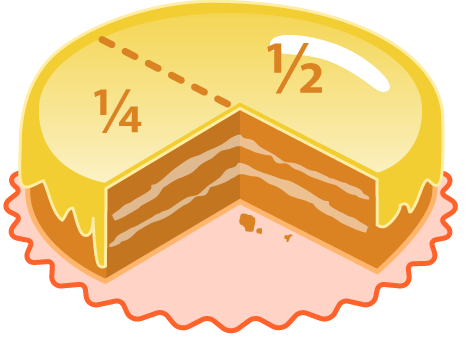# Trigonometry with Fractional Part? Too Much!

Calculus Level 4$\dfrac {\left ( \tan \left ( \{x\} - 1 \right ) \right ) \sin \{ x \} }{ \{ x \} \left ( \{ x \} - 1 \right ) }$

What is the value of the above expression if we take for an arbitrary small value of $x$? Which is to say, what is the limit of the said expression when $x$ approaches $0$?

Details and assumptions:

• $\{ A \}$ denote the fractional part of $A$. For example, $A = 1.74 \Rightarrow \{ A \} = 0.74$, $B = -1.3 \Rightarrow \{ B \} = 0.7$

• $\{x\}=x-\lfloor x \rfloor$

###### Image Credit: Wikipedia Fraction
×

Problem Loading...

Note Loading...

Set Loading...